# 机器学习技法笔记(6)-RBF Network(径向基函数网络)

2017.3.3更新: TensorFlow版本KMeans与RBFNet

2017.2.28更新：Python实现RBFNet

$RBF(x,c)=exp\left( - \frac{(x-c)^2}{r^2}\right)$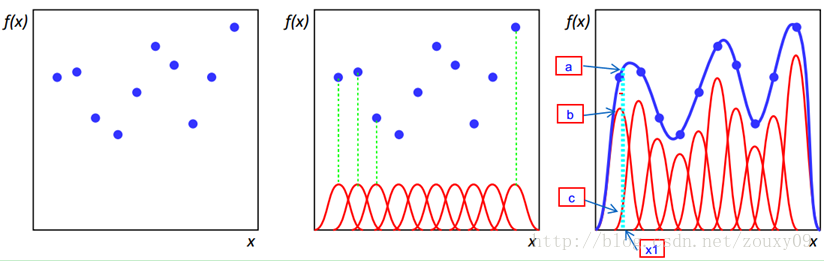## RBF Network

$h(\mathbf{x}) = Output\left( \sum\limits_{m=1}^M\beta_mRBF(\mathbf{x}, \mu_m)\right)$

RBF Network与普通神经网络有什么联系区别呢？如下: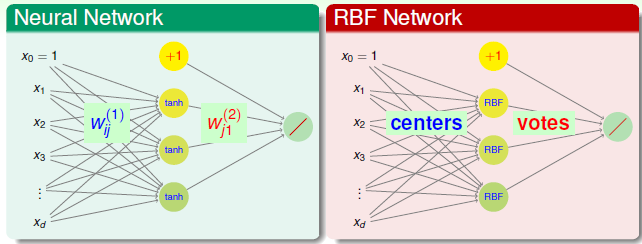## 几种形式

RBF的隐层神经元也就是center的选择是个很关键的问题，因为只有中心确定了之后，RBF函数才能够确定。下面基于不同的中心介绍两种类型的RBF。

### Full RBF Network

Full RBF Network，顾名思义便是所有的数据节点都作为中心。

$h(\mathbf{x}) = Output\left( \sum\limits_{m=1}^M\beta_mRBF(\mathbf{x}, \mu_m)\right)$

$M=N, \mu_m=\mathbf{x}_m$, 这样的话，预测新的数据点，需要计算该点与所有训练数据点的距离，也就是相似度，然后结合权重$\beta$进行线性组合，此过程就是将所有的训练数据点对预测点的影响聚集到一起，距离越近，影响越大，这样得到最终的结果。比如用均匀影响做分类的话，即$\beta_m =1 \cdot y_m$,即:

$g(\mathbf{x}) = sign \left( \sum\limits_{m=1}^Ny_m \exp\left( -\gamma ||\mathbf{x}-\mathbf{x}_m||^2\right)\right)$

### 空间转换

$h(\mathbf{x}) = \sum\limits_{m=1}^M\beta_mRBF(\mathbf{x}, \mathbf{x}_m)$

$\mathbf{z}_n = [RBF(\mathbf{x}_n, \mu_1), RBF(\mu_n, \mathbf{x}_2), \cdots, RBF(\mathbf{x}_n, \mu_M)]$

$h(\mathbf{z}) = \sum\beta_m\mathbf{z}_m$

## K-Means

$g_{svm}(\mathbf{x})= sign\left( \sum\limits_{SV}\alpha_n y_n\exp(-\gamma||\mathbf{x}-\mathbf{x}_n||^2)\right)$

$\mathbf{x}_1,\mathbf{x}_2 \in S_m \Leftrightarrow \mu_m \approx \mathbf{x_1} \approx \mathbf{x_2}$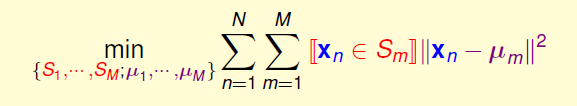$\arg\min\limits_{m}||\mathbf{x}_n - \mu_m||^2$

$E_{in} = \min\limits_{u_m} \sum\limits_{\mathbf{x}_n \in S_m}||\mathbf{x}_n-\mu_m||^2$

\begin{align}\frac{\partial E_{in}}{\partial \mu_m} &= -2\left( \sum\limits_{\mathbf{x}_n \in S_m}\mathbf{x}_n - \mu_m\right)\\&=-2\left(\left( \sum\limits_{\mathbf{x}_n \in S_m}\mathbf{x}_n\right) - |S_m|\mu_m\right)=0 \\ \mu_m &=\frac{\sum\limits_{\mathbf{x}_n \in S_M} \mathbf{x}_n}{|S_m|}\end{align}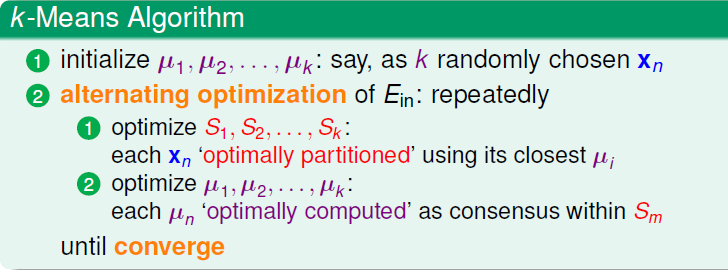• K的选择，一般需要一些先验知识，影响很大
• $\mu$ 初始化很关键，对整个收敛速度与质量影响很大，需要多测试
• 收敛性，K-Means可以一定收敛，因为交替更新的两个过程都是在使得$E_{in}$下降。不过由于$E_{in}$ 并不是凸函数，因此我们得到可能不是全局最优解。
• K-Means属于无监督的特征转换过程，类似与AutoEncoder。

## RBF Net With K-Means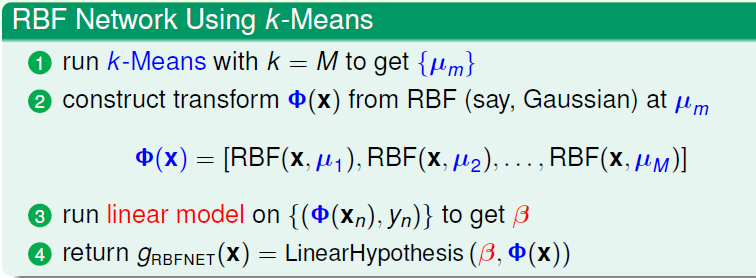RBF Net的超参数只有三种: $M$(中心个数)，RBF函数中的，比如高斯里面的$\gamma$，以及权重。因此RBFNet是一种简单，快速的逼近算法，可以用于回归，分类，其中做函数逼近更多一些，下一节重点介绍训练以及实现。

## 实现

### RBFNet

\begin{align}h(\mathbf{x}) &= \sum\limits_{m=1}^M\beta_mRBF(\mathbf{x}, \mu_m) \\ &=\sum\limits_{m=1}^M\beta_m * \exp(-\gamma_m * ||\mathbf{x}-\mathbf{c}||^2) \end{align}

• 计算出每个簇内的发散平均程度，即距离中心的平均距离: $\sigma = \frac{1}{m}\sum||\mathbf{x}-\mu_m||$
• $\gamma_m = \frac{1}{2\sigma^2}$

• 使用Linear Regression就可以直接解出$\beta$:$\beta = (\mathbf{Z^TZ})^{-1}\mathbf{Z}^T\mathbf{y}$，其中$\mathbf{Z}$是所有训练数据的rbf的结果，$\mathbf{y}$是所有训练数据的y。
• 按照梯度下降的方式来训练参数(只在TensorFlow版本实现)

### 结果

CPU版本：

1. 使用$y=sum(x)$的3000条数据，逼近结果如下:
1. 使用$y=2x$的3000条数据，逼近效果如下:

GPU版本结果:

1. 使用$y=sum(x)$的3000数据:

2. 使用$y=2x$的3000条训练数据:

1. Linear Regression方式:

2. 梯度下降训练方式:

Numpy版KMeans+RBFNet 与 TensorFlow版KMeans+RBFNet的完整代码: RBFNet With K-Means